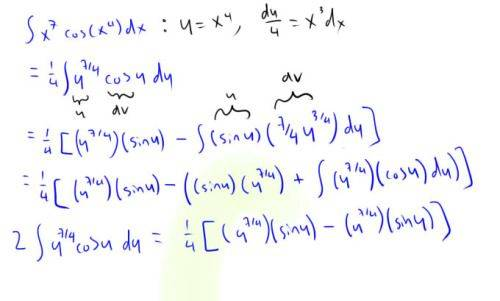# Substitution and Integration by Parts

## Homework Statement

First make a substitution and then use integration by parts to evaluate the integral.

∫x$^{7}$cos(x$^{4}$)dx

## Homework Equations

Equation for Substitution: ∫f(g(x))g'(x)dx = ∫f(u)du
Equation for Integration by Parts: ∫udv = uv - ∫vdu

## The Attempt at a Solution

So here's my attempted solutionI made a substitution and tried using integration by parts twice but I got stuck on the last line since it turns out to be zero... I know I went wrong somewhere but I can't seem to find my mistake. Any help would be really appreciated! Thanks :)

## Answers and Replies

CAF123
Gold Member
The second line is incorrect - when you made the u substitution you did not use your expression for dx in terms of du.

•1 person
HallsofIvy
Science Advisor
Homework Helper
Start by writing the integral as $\int x^4cos(x^4)(x^3dx)$ and it is clearer.

•1 person
I see my mistake now! Thanks for the help :)